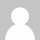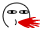# 假期，所剩无几

``\$(pwd)``

``\$(cd "\$(dirname "\$0")"; pwd)``

``\$(cd "\$(dirname "\${BASH_SOURCE}")"``

``count(\$a) – count(array_intersect(\$a, \$b));``

``count(array_diff(\$a, \$b));``

``````function () {
return \$a, \$b, \$c;
}``````

``````function abc() {
return array(\$a, \$b, \$c);
}
list(\$x, \$y, \$z) = abc();``````

``````function abc() {
\$a = array();
\$a[] = 1;
\$a[] = 2;
\$b = 11;
\$c = 12;
return array(\$a, \$b, \$c);
}
list(\$x, \$y, \$z) = abc();
var_dump(\$x, \$y, \$z);``````

``````function make_ranked(\$rank) {
\$last = substr( \$rank, -1 );
\$seclast = substr( \$rank, -2, -1 );
if( \$last > 3 || \$last == 0 ) \$ext = 'th';
else if( \$last == 3 ) \$ext = 'rd';
else if( \$last == 2 ) \$ext = 'nd';
else \$ext = 'st';
if( \$last == 1 && \$seclast == 1) \$ext = 'th';
if( \$last == 2 && \$seclast == 1) \$ext = 'th';
if( \$last == 3 && \$seclast == 1) \$ext = 'th';
return \$rank.\$ext;
}``````

``````function getTinyUrl(\$url) {
return file_get_contents("http://tinyurl.com/api-create.php?url=".\$url);
}``````Tags: , ,

1.我发现你的人生才是充实的。

1.MOD回复

@耳朵的主人: 额，言重了大家都是充实的

2.@kn007: 我总觉得我是在不停的瞎忙碌，有时候连自己到底做什么都不知道。

3.MOD回复

@耳朵的主人: 没办法，人生必须经历的

2.LV1回复

早就没有假期了

1.MOD回复

@鲜活: